• +91 9971497814
• info@interviewmaterial.com

# RD Chapter 12- Some Applications of Trigonometry Ex-12.1 Interview Questions Answers

### Related Subjects

Question 1 : A tower stands vertically on the ground. From a point on the ground, 20 m away from the foot of the tower, the angle of elevation of the top of the tower is 60°. What is the height of the tower ?

Answer 1 : Let TS is the tower and P is a point which is 20 m away from the foot of the tower and angle of elevation of T is 60°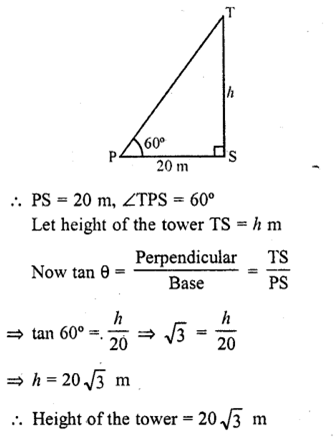Question 2 : The angle of elevation of a ladder leaning against a wall is 60° and the foot of the ladder is 9.5 m away from the wall. Find the length of the ladder.

Answer 2 : Let LM is the ladder which makes an angle of 60° with the wall LM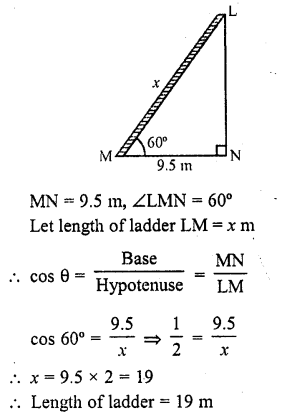Question 3 : A ladder is placed along a wall of a house such that its upper end is touching the top of the wall. The foot of the ladder is 2 m away from the wail and the ladder is making an angle of 60° with the level of the ground. Determine the height of the wall.

Answer 3 : Let LM be the ladder which makes an angle of 60° with the wall LN and at a distance of 2 m from the foot of the wall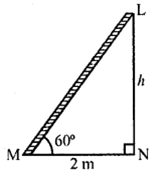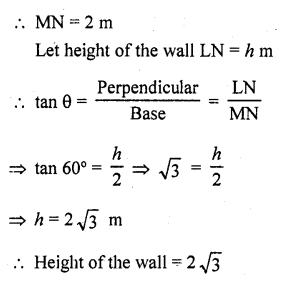Question 4 : An electric pole is 10 m high. A steel wire tied to top of the pole is affixed at a point on the ground to keep the pole up right. If the wire makes an angle of 45° with the horizontal through the foot of the pole, find the length of the wire.

Answer 4 : Let AB be the pole and a wire AC is tied to the top of the pole with a point C on the ground which makes an angle of 45° with the ground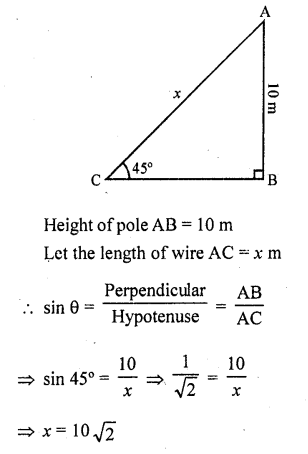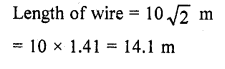Question 5 : A kite is flying at a height of 75 metres from the ground level, attached to a string inclined at 60° to the horizontal. Find the length of the string to the nearest metre.

Let K be the kite flying in the sky at a height of 75 m from the ground LM. The string KL makes an angle of 60° to the ground
Let length of string KL = x m
KM = 75 m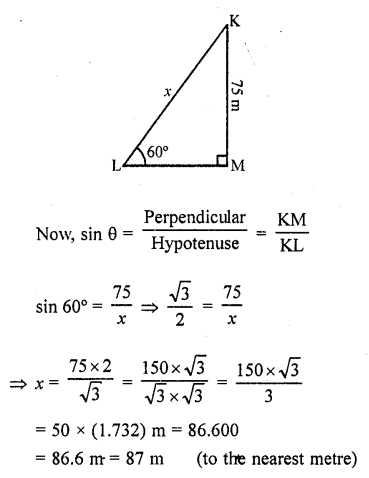Question 6 : A ladder 15 m long just reaches the top of a vertical wall. If the ladders makes an angle of 60° with the wall, then find the height of the wall.

Given that, the height of the ladder = 15 m
Let the height of the vertical wall = h
and the ladder makes an angle of elevation 60° with the wall i.e., θ = 60°.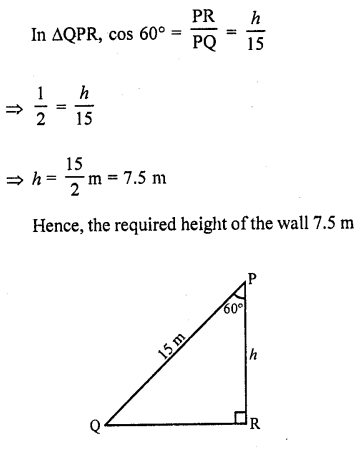Question 7 : A vertical tower stands on a horizontal plane and is surmounted by a vertical flag-staff. At a point on the plane 70 metres away from the tower, an observer notices that the angles of elevation of the top and the bottom of the flag-staff are respectively 60° and 45°. Find the height of the flag-staff and that of the tower.

Answer 7 : Let TR be the tower and FT is the flag on it. P is an point on the ground 70 m away from the foot of the tower. From P, the angle of elevation of the top and bottom of the flag are 60° and 45° respectively Let h be the height of flag staff and x be the height of the tower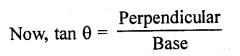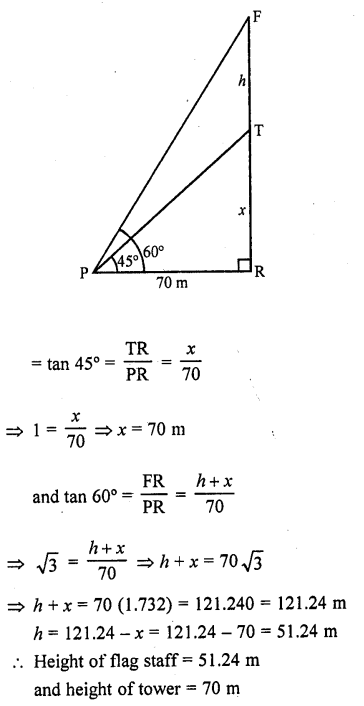Question 8 : A vertically straight tree, 15 m high, is broken by the wind in such a way that its top just touches the ground and makes an angle of 60° with the ground. At what height from the ground did the tree break ? [CBSE 1995]

Let TR be the tree whose height is 15 m
Let it is broken from A and its top T touches the ground at B making an angle of 60° with the ground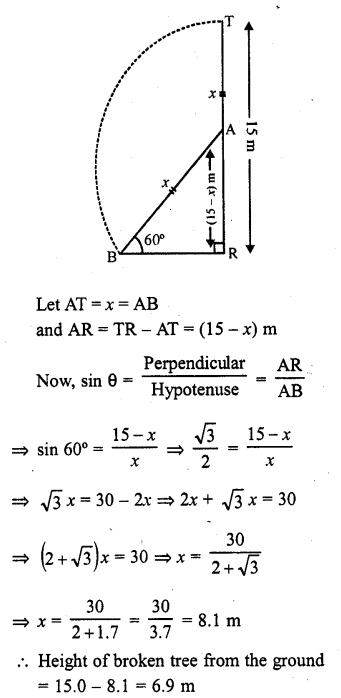Question 9 : A vertical tower stands on a horizontal plane and is surmounted by a vertical flag-staff of height 5 metres. At a point on the plane, the angles of elevation of the bottom and the top of the flag-staff are respectively 30° and 60°. Find the height of the tower. (C.B.S.E. 1995)

Answer 9 : Let TR be the tower and FT is a flag staff on it. A is a point on the ground such that it makes angles of elevation of the bottom and top of the flag staff of 30° and 60° respectively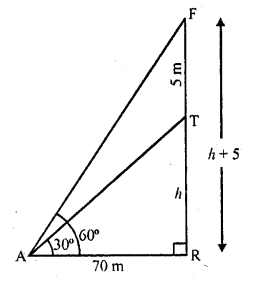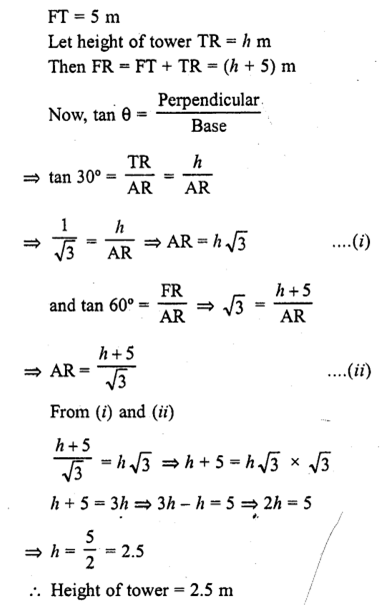Question 10 : A person observed the angle of elevation of the top of a tower as 30°. He walked 50 m towards the root of the tower along level ground and found the angle of elevation of the top of the tower as 60°. Find the height of the tower.

Answer 10 : Let TR be the tower. A person at A on the ground observes the angle of elevation of the top T of the tower as 30° and then moves towards the foot of the tower. At a distance of 50 m at B, the angle of elevation becomes 60°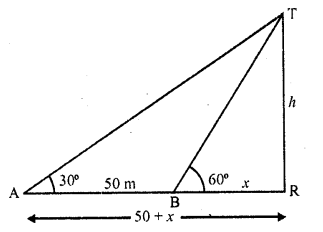Let h be the height of the tower and BR = x, then AR = (50 + x)